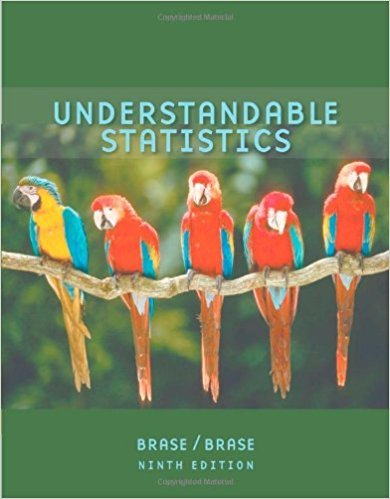×
Get Full Access to Statistics - Textbook Survival Guide
Get Full Access to Statistics - Textbook Survival Guide

×

Textbooks / Statistics / Understandable Statistics 9

# Understandable Statistics 9th Edition - Solutions by Chapter## Full solutions for Understandable Statistics | 9th Edition

ISBN: 9780618949922Understandable Statistics | 9th Edition - Solutions by Chapter

Solutions by Chapter
4 5 0 433 Reviews
##### ISBN: 9780618949922

This expansive textbook survival guide covers the following chapters: 57. Understandable Statistics was written by and is associated to the ISBN: 9780618949922. Since problems from 57 chapters in Understandable Statistics have been answered, more than 115274 students have viewed full step-by-step answer. This textbook survival guide was created for the textbook: Understandable Statistics, edition: 9. The full step-by-step solution to problem in Understandable Statistics were answered by , our top Statistics solution expert on 01/04/18, 09:09PM.

Key Statistics Terms and definitions covered in this textbook
• Additivity property of x 2

If two independent random variables X1 and X2 are distributed as chi-square with v1 and v2 degrees of freedom, respectively, Y = + X X 1 2 is a chi-square random variable with u = + v v 1 2 degrees of freedom. This generalizes to any number of independent chi-square random variables.

• Adjusted R 2

A variation of the R 2 statistic that compensates for the number of parameters in a regression model. Essentially, the adjustment is a penalty for increasing the number of parameters in the model. Alias. In a fractional factorial experiment when certain factor effects cannot be estimated uniquely, they are said to be aliased.

• Backward elimination

A method of variable selection in regression that begins with all of the candidate regressor variables in the model and eliminates the insigniicant regressors one at a time until only signiicant regressors remain

• Conditional probability density function

The probability density function of the conditional probability distribution of a continuous random variable.

• Conidence coeficient

The probability 1?a associated with a conidence interval expressing the probability that the stated interval will contain the true parameter value.

• Conidence interval

If it is possible to write a probability statement of the form PL U ( ) ? ? ? ? = ?1 where L and U are functions of only the sample data and ? is a parameter, then the interval between L and U is called a conidence interval (or a 100 1( )% ? ? conidence interval). The interpretation is that a statement that the parameter ? lies in this interval will be true 100 1( )% ? ? of the times that such a statement is made

• Continuity correction.

A correction factor used to improve the approximation to binomial probabilities from a normal distribution.

• Convolution

A method to derive the probability density function of the sum of two independent random variables from an integral (or sum) of probability density (or mass) functions.

• Covariance

A measure of association between two random variables obtained as the expected value of the product of the two random variables around their means; that is, Cov(X Y, ) [( )( )] =? ? E X Y ? ? X Y .

• Critical value(s)

The value of a statistic corresponding to a stated signiicance level as determined from the sampling distribution. For example, if PZ z PZ ( )( .) . ? =? = 0 025 . 1 96 0 025, then z0 025 . = 1 9. 6 is the critical value of z at the 0.025 level of signiicance. Crossed factors. Another name for factors that are arranged in a factorial experiment.

• Crossed factors

Another name for factors that are arranged in a factorial experiment.

• Density function

Another name for a probability density function

• Discrete distribution

A probability distribution for a discrete random variable

• Distribution free method(s)

Any method of inference (hypothesis testing or conidence interval construction) that does not depend on the form of the underlying distribution of the observations. Sometimes called nonparametric method(s).

• Error mean square

The error sum of squares divided by its number of degrees of freedom.

• Extra sum of squares method

A method used in regression analysis to conduct a hypothesis test for the additional contribution of one or more variables to a model.

• F distribution.

The distribution of the random variable deined as the ratio of two independent chi-square random variables, each divided by its number of degrees of freedom.

• False alarm

A signal from a control chart when no assignable causes are present

• Fraction defective control chart

See P chart

• Frequency distribution

An arrangement of the frequencies of observations in a sample or population according to the values that the observations take on